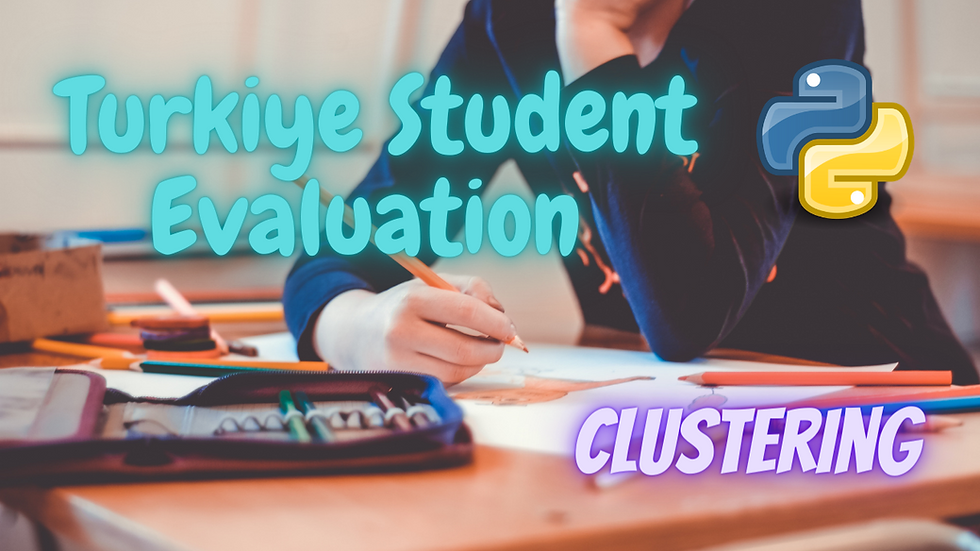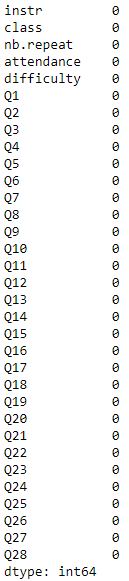top of page
• Hackers Realm

# Turkiye Student Evaluation Analysis using Python | Clustering | Machine Learning Project Tutorial

Updated: Jun 4

Discover insights from Turkiye student evaluations using Python! This tutorial covers clustering techniques to analyze and understand student feedback. Learn how to preprocess data, apply clustering algorithms, and evaluate their performance. Dive into machine learning with Python and unlock valuable insights from educational data. Join this tutorial to master the art of student evaluation analysis and enhance decision-making in educational settings. #TurkiyeStudentEvaluation #Python #Clustering #MachineLearning #DataAnalysis #EducationalDataAnalysisTurkiye Student Evaluation - Clustering

In this project tutorial, we will learn Turkiye student evaluation analysis using python. Furthermore, we will discuss unsupervised learning, principal component analysis, clustering, elbow method and dendrogram.

You can watch the video-based tutorial with step by step explanation down below.

## Dataset Information

This data set contains a total of 5820 evaluation scores provided by students from Gazi University in Ankara (Turkey). There is a total of 28-course specific questions and additional 5 attributes.

#### Attribute Information:

instr: Instructor's identifier; values taken from {1,2,3} class: Course code (descriptor); values taken from {1-13} repeat: Number of times the student is taking this course; values are taken from {0,1,2,3,...} attendance: Code of the level of attendance; values from {0, 1, 2, 3, 4} difficulty: Level of difficulty of the course as perceived by the student; values taken from {1,2,3,4,5} Q1: The semester course content, teaching method and evaluation system were provided at the start. Q2: The course aims and objectives were clearly stated at the beginning of the period. Q3: The course was worth the amount of credit assigned to it. Q4: The course was taught according to the syllabus announced on the first day of class. Q5: The class discussions, homework assignments, applications and studies were satisfactory. Q6: The textbook and other courses resources were sufficient and up to date. Q7: The course allowed fieldwork, applications, laboratory, discussion and other studies. Q8: The quizzes, assignments, projects and exams contributed to help the learning. Q9: I greatly enjoyed the class and was eager to actively participate during the lectures. Q10: My initial expectations about the course were met at the end of the period or year. Q11: The course was relevant and beneficial to my professional development. Q12: The course helped me look at life and the world from a new perspective. Q13: The Instructor's knowledge was relevant and up to date. Q14: The Instructor came prepared for classes. Q15: The Instructor taught in accordance with the announced lesson plan. Q16: The Instructor was committed to the course and was understandable. Q17: The Instructor arrived on time for classes. Q18: The Instructor has a smooth and easy to follow delivery/speech. Q19: The Instructor made effective use of class hours. Q20: The Instructor explained the course and was eager to be helpful to students. Q21: The Instructor demonstrated a positive approach to students. Q22: The Instructor was open and respectful of the views of students about the course. Q23: The Instructor encouraged participation in the course. Q24: The Instructor gave relevant homework assignments/projects, and helped/guided students. Q25: The Instructor responded to questions about the course inside and outside of the course. Q26: The Instructor's evaluation system (midterm and final questions, projects, assignments, etc.) effectively measured the course objectives. Q27: The Instructor provided solutions to exams and discussed them with students. Q28: The Instructor treated all students in a right and objective manner.

Q1-Q28 are all Likert-type, meaning that the values are taken from {1,2,3,4,5}

## Import modules

First, we have to import all the basic modules we will be needing for this project.

```import pandas as pd
import numpy as np
import seaborn as sns
import matplotlib.pyplot as plt
import warnings
%matplotlib inline
warnings.filterwarnings('ignore')
pd.options.display.max_columns = 99```
• pandas - used to perform data manipulation and analysis

• numpy - used to perform a wide variety of mathematical operations on arrays

• matplotlib - used for data visualization and graphical plotting

• seaborn - built on top of matplotlib with similar functionalities

• %matplotlib - to enable the inline plotting

• warnings - to manipulate warnings details

• filterwarnings('ignore') is to ignore the warnings thrown by the modules (gives clean results)

• max_columns - sets the maximum number of columns displayed when a dataframe is displayed.

```df = pd.read_csv("turkiye-student-evaluation_generic.csv")Turkiye Student Evaluation Dataset
• We have question values from 1 to 28 questions.

```# statistical info
df.describe()```Statistical Information of Dataset
• The mean value of the questions are 3.

• You can plot a graph using the ranges and analyze it further.

```# datatype info
df.info()```Datatype Information
• All the datatype values are integer (int64).

• If a dataset contains millions of data, then the memory usage will be large. Similarly, you can use int8 instead of int64.

• Reducing the data type for all the columns will lower memory usage.

## Preprocessing the dataset

Let us check for NULL values in the dataset.

```# check for null values
df.isnull().sum()```Count of NULL Values in Dataset
• There are no NULL values in the dataset.

## Exploratory Data Analysis

Let us explore the columns.

```# set new style for the graph
plt.style.use("fivethirtyeight")```
`sns.countplot(df['instr'])`Distribution of Instructors
• Instructor 3 had taken more courses compared to instructors 1 and 2.

`sns.countplot(df['class'])`Distribution of Students in Class
• It shows the number of students in that particular class.

Let us find the mean of the questions.

```# find mean of questions
x_questions = df.iloc[:, 5:33]
q_mean = x_questions.mean(axis=0)
total_mean = q_mean.mean()```
```q_mean = q_mean.to_frame('mean')
q_mean.reset_index(level=0, inplace=True)• We converted the question mean into a data frame.

`total_mean`• It implies that the average rating for the questions is 3.

Let us plot these mean values in terms of a graph.

```plt.figure(figsize=(14,7))
sns.barplot(x='index', y='mean', data=q_mean)```• Similarly, you can plot a graph of other features by converting it into a data frame.

## Correlation Matrix

A correlation matrix is a table showing correlation coefficients between variables. For this project, all are input attributes with no output attributes.

```corr = df.corr()
plt.figure(figsize=(18,18))
sns.heatmap(corr, annot=True, cmap='coolwarm')```Correlation Matrix
• The warm color shows a positive correlation, and the cool color is a negative correlation.

• In supervised learning, we can drop highly correlated attributes.

• Since this is unsupervised learning, we will reduce the dimension of the dataset using principal component analysis.

## Principal component analysis

The principal component analysis reduces the dimensionality of large data sets by transforming a large group of variables into a smaller one that still contains most of the information in the large set. This method reduces the computational time to train the model and it will also retain the information of the data set.

`X = df.iloc[:, 5:33]`
```from sklearn.decomposition import PCA
pca = PCA(n_components=2, random_state=42)
X_pca = pca.fit_transform(X)```
`X_pca`• Using the fit_tranform() function allows us to fit the dataset in the model and transform the inputs based on the number of components.

• As a result, we get two columns with all the samples.

```# how much info we retained from the dataset
pca.explained_variance_ratio_.cumsum()```• We have retained 86% of the original information from the dataset.

• We have to retain 95% of the original data for best practices. However, we have to visualize the information, so we will use 86% information for this specific project.

## Model Training

Let us initiate the clustering mechanism using Kmeans and Elbow methods.

```# Kmeans clustering
from sklearn.cluster import KMeans
distortions = []
cluster_range = range(1,6)

# elbow method
for i in cluster_range:
model = KMeans(n_clusters=i, init='k-means++', n_jobs=-1, random_state=42)
model.fit(X_pca)
distortions.append(model.inertia_)```
• Distortion is the sum of square distances from each point to its assigned center.

• We will pick the no. of clusters with optimal distortion.

• n_jobs relates to parallel processing. Stating n-jobs=-1 will use all the cores in our CPU to process the model.

• model.fit() is used to fit the data.

• Afterwards, the distorted values will append to the distortions.

After running the model let us plot the distortions.

```plt.plot(cluster_range, distortions, marker='o')
plt.xlabel("Number of clusters")
plt.ylabel('Distortions')
plt.show()```Elbow Method
• We use elbow methods to find the number of clusters.

• The shape in a graph represents an elbow.

• We take the best cluster number from the joint of the elbow.

• The best cluster appears to be 3.

Let us now use cluster 3.

```# use best cluster
model = KMeans(n_clusters=3, init='k-means++', n_jobs=-1, random_state=42)
model.fit(X_pca)
y = model.predict(X_pca)```
```plt.scatter(X_pca[y==0, 0], X_pca[y==0, 1], s=50, c='red', label='cluster 1')
plt.scatter(X_pca[y==1, 0], X_pca[y==1, 1], s=50, c='yellow', label='cluster 2')
plt.scatter(X_pca[y==2, 0], X_pca[y==2, 1], s=50, c='green', label='cluster 3')
plt.scatter(model.cluster_centers_[:,0], model.cluster_centers_[:, 1], s=100, c='blue', label='centroids')
plt.title('Cluster of students')
plt.xlabel('PCA 1')
plt.ylabel('PCA 2')
plt.legend()
plt.show()```• The operation X_pca[y==0, 0] means that it will filter only the rows with cluster label 0 and the second is a column number.

• We have three clusters from the dataset.

• The blue centroid is the center of the cluster.

• The new point introduced will be assigned to the nearest cluster.

• We can provide an output variable for each cluster. For example, The output variable red indicates that the students are less satisfied. If the output variable is in green, it implies that the students are in a neutral state. And for yellow, the students are satisfied.

Let us see how many points belong to each cluster.

```from collections import Counter
Counter(y)```• 0 belongs to red, 1 belongs to yellow and 2 belongs to green.

Training the model for the entire dataset.

```model = KMeans(n_clusters=3, init='k-means++', n_jobs=-1, random_state=42)
model.fit(X)
y = model.predict(X)```
`Counter(y)`• Even after training with 28 dimensions, there is no significant difference in the output.

• Therefore, we can train it with only two dimensions.

#### Dendrogram.

Let's use a dendrogram to find the number of clusters.

```# dendogram
import scipy.cluster.hierarchy as hier
plt.title('Dendogram')
plt.xlabel("Questions")
plt.ylabel("Distance")
plt.show()```Dendogram
• Each combining part is considered a cluster. Later on, all the clusters combined to form a single cluster.

• We observe two major clusters of colors, red and yellow.

• In the dendrogram, cluster 2 of the color yellow appears to be the best cluster.

We will process cluster 2 using Agglomerative Clustering.

```from sklearn.cluster import AgglomerativeClustering
y = model.fit_predict(X_pca)```
```plt.scatter(X_pca[y==0, 0], X_pca[y==0, 1], s=50, c='red', label='cluster 1')
plt.scatter(X_pca[y==1, 0], X_pca[y==1, 1], s=50, c='yellow', label='cluster 2')
plt.title('Cluster of students')
plt.xlabel('PCA 1')
plt.ylabel('PCA 2')
plt.legend()
plt.show()```• model.fit_predict() is to train and predict the cluster label.

• Yellow represent the dissatisfied and Red represents the satisfied.

Let us see how many points belong to each cluster.

`Counter(y)`• We have predicted the output and displayed them in form of a graph.

• This concludes our analysis of the dataset.

## Final Thoughts

• You can use different hyperparameters to obtain different results.

• You need to find the best number of clusters based on the requirements.

In this article, we have analyzed Turkiye student evaluation using machine learning. We used clustering in the data set. Likewise, we discussed unsupervised learning and principal component analysis.

Get the project notebook from here